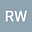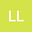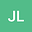Dynamical transition for a 3-component Lotka-Volterra Model with Diffusion
•••• Ruili Wu,
• Limei Li,
• Junyan LI
Ruili Wu
Jincheng College of Sichuan University

Corresponding Author:[email protected]

Author ProfileLimei Li
Sichuan Normal University
Author ProfileJunyan LI
Jincheng college of Sichuan University
Author Profile## Abstract

The main objective of this article is to investigate the dynamical transition for a 3-component Lotka-Volterra model with diffusion. Based on the spectral analysis, the principle of exchange of stability conditions for eigenvalues are obtained. In addition, when $\delta_0<\delta_1$, the first eigenvalues are complex, and we show that the system undergoes a continuous or jump transition. In the small oscillation frequency limit, the transition is always continuous and the time periodic rolls are stable after the transition. In the case where $\delta_0>\delta_1$, the first eigenvalue is real. Generically, the first eigenvalue is simple and all three types of transition are possible. In particular, the transition is mixed if $\int_{\Omega}e_{k_0}^3dx\neq 0$, and is continuous or jump in the case where $\int_{\Omega}e_{k_0}^3dx= 0$. In this case we also show that the system bifurcates to two saddle points on $\delta<\delta_1$ as $\tilde{\theta}> 0$, and bifurcates to two stable singular points on $\delta>\delta_1$ as $\tilde{\theta}< 0$ where $\tilde{\theta}$ depends on the system parameters.